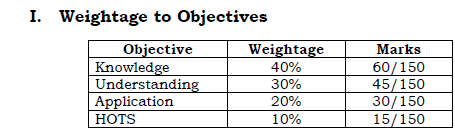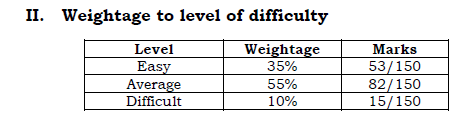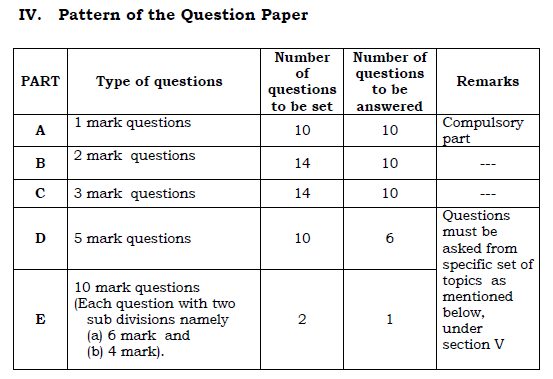# Karnataka Board 1st PUC Maths Blueprint

Students who are preparing for the KSEEB 1st PUC exams can get an idea about the exam pattern and question format by downloading the Karnataka Board 1st PUC Maths Blueprint. It will help them enhance their exam preparation. In the Blueprints, students get an overview as to which chapter of their books will be allocated how many marks in final papers.

Students can access the Karnataka Board 1st PUC Maths Blueprint from the link given:

## Karnataka Board 1st PUC Maths Question Paper Design

The 3 hour 15 min question paper is of 100 marks. The weightage of the distribution of marks over different dimensions of the question paper is given below:III. Weightage to content

 Chapter No. Content Teaching Hours Marks 1 SETS 8 8 2 RELATIONS AND FUNCTIONS 10 11 3 TRIGONOMETRIC FUNCTIONS 18 19 4 PRINCIPLE OF MATHEMATICAL INDUCTION 4 5 5 COMPLEX NUMBERS AND QUADRATIC EQUATIONS 8 9 6 LINEAR INEQUALITIES 8 7 7 PERMUTATION AND COMBINATION 9 9 8 BINOMIAL THEOREM 7 8 9 SEQUENCE AND SERIES 9 11 10 STRAIGHT LINES 10 10 11 CONIC SECTIONS 9 9 12 INTRODUCTION TO 3D GEOMETRY 5 7 13 LIMITS AND DERIVATIVES 14 15 14 MATHEMATICAL REASONING 6 6 15 STATISTICS 7 7 16 PROBABILITY 8 9 Total 140 150

### Pattern of Question Paper

The question paper pattern provided follows the given instructions.

Part A : 10 compulsory questions each carrying 1 mark;

Part B : 10 questions to be answered out of 14 questions each

carrying 2 marks ;

Part C : 10 questions to be answered out of 14 questions each

carrying 3 marks;

Part D: 6 questions to be answered out of 10 questions each

carrying 5 marks;

Part E : 1 question to be answered out of 2 questions each carrying

10 marks with subdivisions (a) and (b) of 6 marks and

4 marks respectively.Questions are taken from the 16 chapters ranging from Sets, Relations and Functions to Linear Equalities, Statistics and Probability.

Stay tuned and get more updates from BYJU’S!